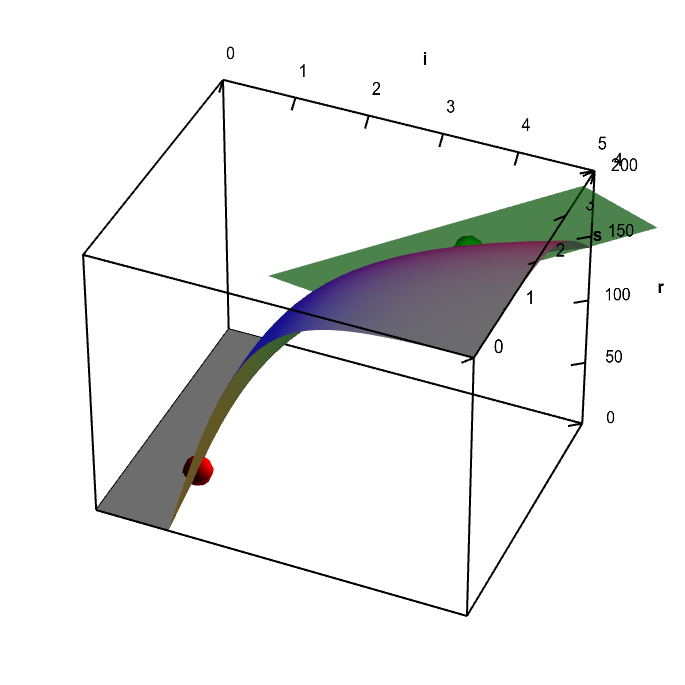# Math Insight

### The multivariable linear approximation

#### The linear approximation in one-variable calculus

The introduction to differentiability in higher dimensions began by reviewing that one variable differentiability is equivalent to the existence of a tangent line. For the neuron firing example of that page, a tangent line of the neuron firing rate $r(i)$ as a function of input $i$ looked like the following figure.

The equation of the tangent line at $i=a$ is \begin{align*} L(i) = r(a) + r'(a)(i-a), \end{align*} where $r '(a)$ is the derivative of $r(i)$ at the point where $i=a$. The tangent line $L(i)$ is called a linear approximation to $r(i)$. The fact that $r(i)$ is differentiable means that it is nearly linear around $i=a$.

Why do we care if $r(i)$ is differentiable? Well, unfortunately, when studying a neuron, the function $r(i)$ may not be a pretty function. In fact, we might not even have a nice equation for $r(i)$. Although it is bad news for mathematicians, neurons don't come with the function $r(i)$ written on them.

In some applications, though, we may know that the input $i$ isn't going to vary a whole lot. We may know that $i$ is going to be close to some value $a$. In that case, we may approximate $r(i)$ by its linear approximation $L(i)$ around $i=a$. If $r(i)$ is differentiable at $i=a$, we make only a small error with this approximation. Moreover, since $L(i)$ is linear, it is easy to work with, much easier than the original $r(i)$. So if we had to do some calculation involving the response of the neuron, we'd make our lives easier by assuming its output rate is $L(i)$ rather than $r(i)$.

#### The linear approximation in two dimensions

The introduction to differentiability in higher dimensions explained that a scalar valued function of two variables is differentiable if and only if it has a tangent plane. For the two-dimensional neuron firing example of that page, we let the neuron firing rate $r(i,s)$ be a function not only of the input $i$ but also of the level of nicotine $s$. A tangent plane of that function calculated a one point looked like the following figure.Neuron firing rate function with tangent plane. A fictitious representation of the firing rate $r(i,s)$ of a neuron in response to an input $i$ and nicotine level $s$. The graph of the function has a tangent plane at the location of the green point, so the function is differentiable there. By rotating the graph, you can see how the tangent plane touches the surface at the that point. You can move the green point anywhere on the surface; as long as it is not along the fold of the graph (where the red point in constrained to be), you can see the tangent plane showing that the function is differentiable. There is no tangent plane to the graph at any point along the fold of the graph (you can move the red point to any point along this fold). The function $r(i,s)$ is not differentiable at any point along the fold. As further evidence of this non-differentiability, the tangent plane jumps to a different angle when you move the green point across the fold.

The equation for the tangent plane at $(i,s) = (a_1,a_2)$ is the expression \begin{align*} L(i,s) = r(a_1,a_2) + \left[\pdiff{r}{i}(a_1,a_2)\right](i-a_1) + \left[\pdiff{r}{s}(a_1,a_2)\right](s-a_2). \end{align*} Just like in the one-variable case, the tangent plane $L(i,s)$ is called a linear approximation to $r(i,s)$. The fact that $r(i,s)$ is differentiable means that it is close to its linear approximation around $(i,s)=\vc{a}$.

We can use the fact that $r(i,s)$ is differentiable to simplify calculations that involve the neural output rate in response to input $i$ and nicotine $s$. For example, looking at the above graph, suppose we wanted to analyze how small changes in nicotine effect the neural response. We might be interested in the response just for input $i$ and nicotine $s$ near the green point. In that case, we could use the equation of the tangent plane. Although it may look complicated, it is actually has a very simple dependence on both $i$ and $s$. Using this linear approximation will be a lot easier than using whatever complicated formula we have for the actual $r(i,s)$. Since $r(i,s)$ isn't differentiable around the red point, we are unable to use a linear approximation there.

Examples of linear approximations can be found in the examples of calculating the derivative.